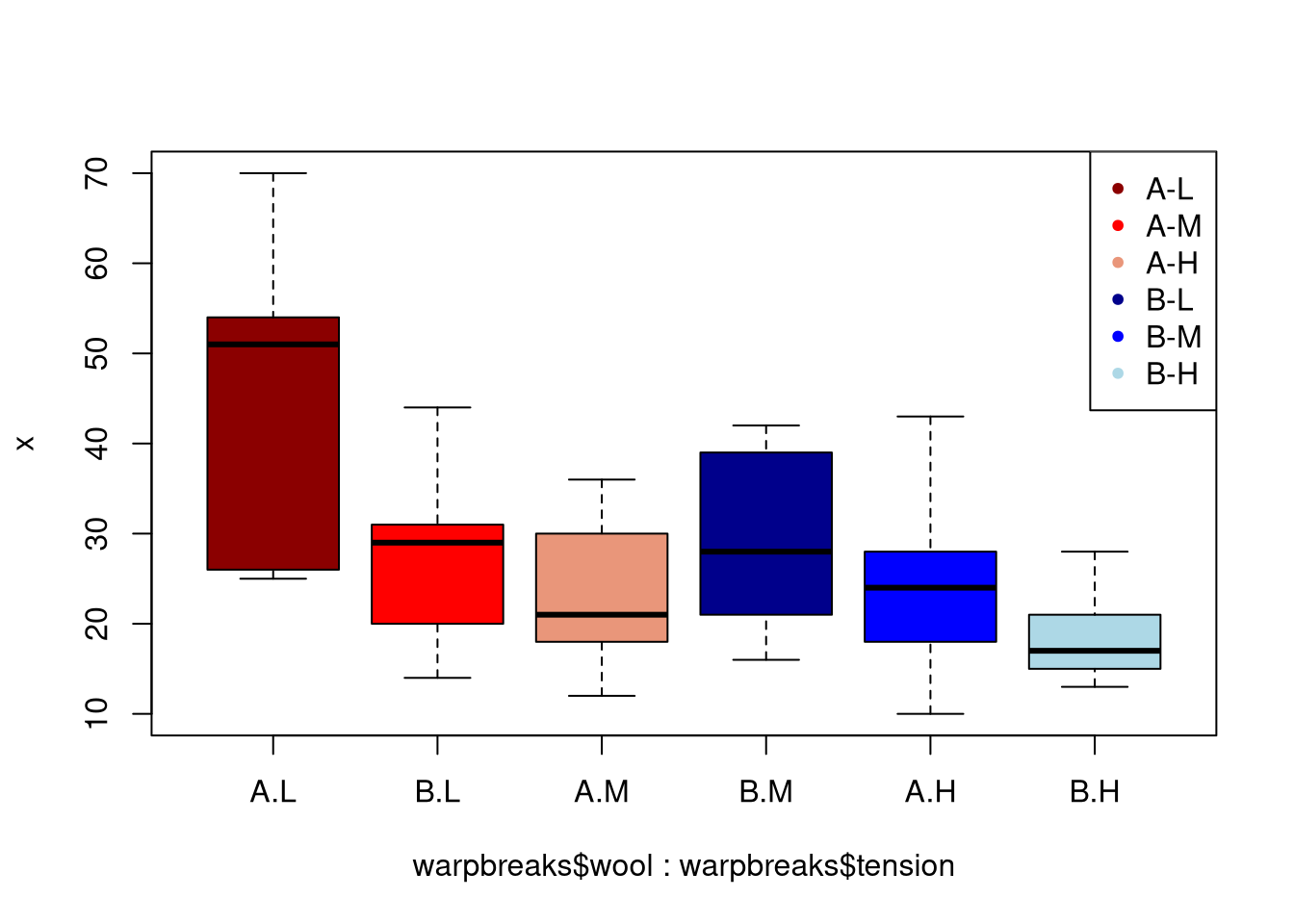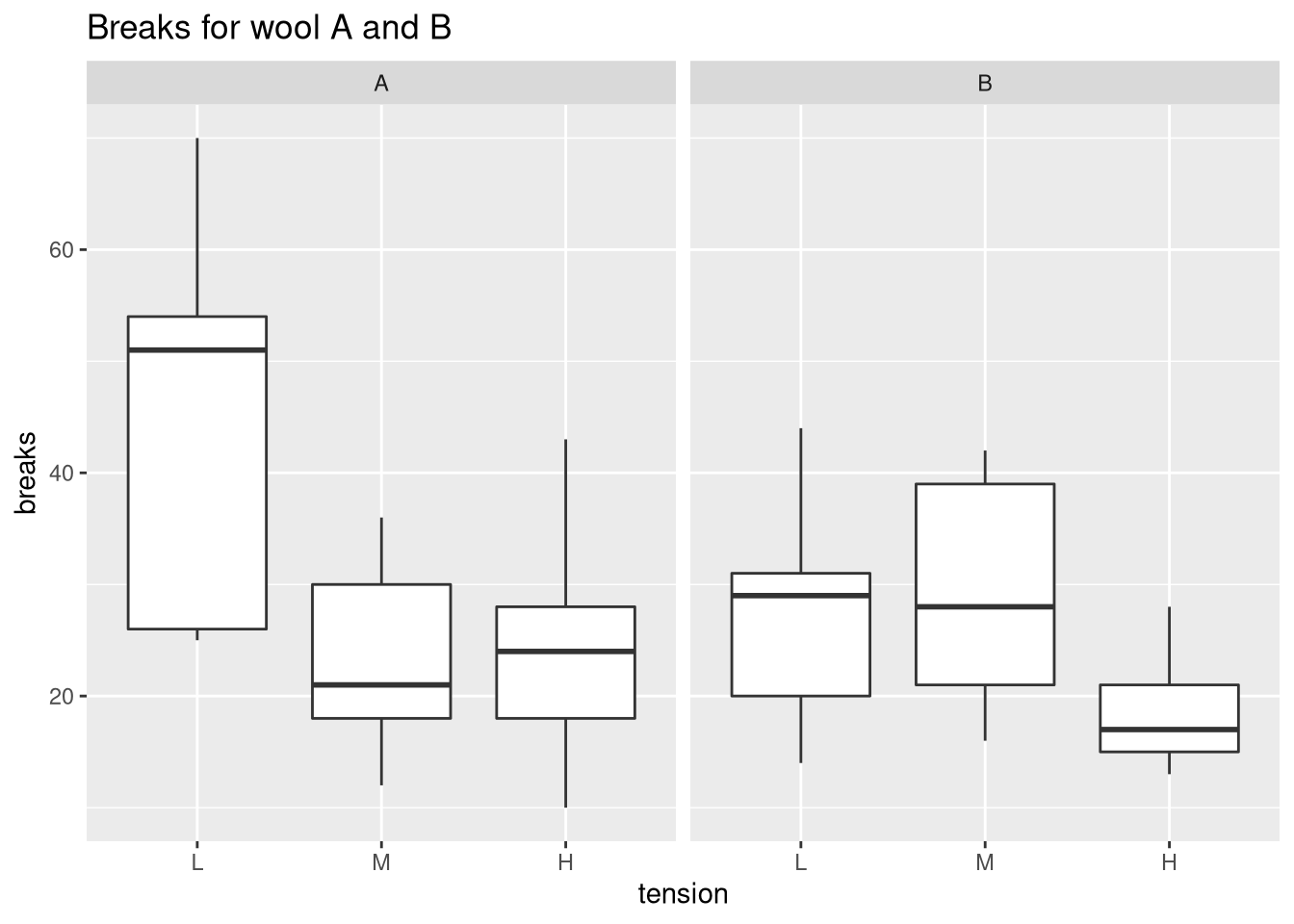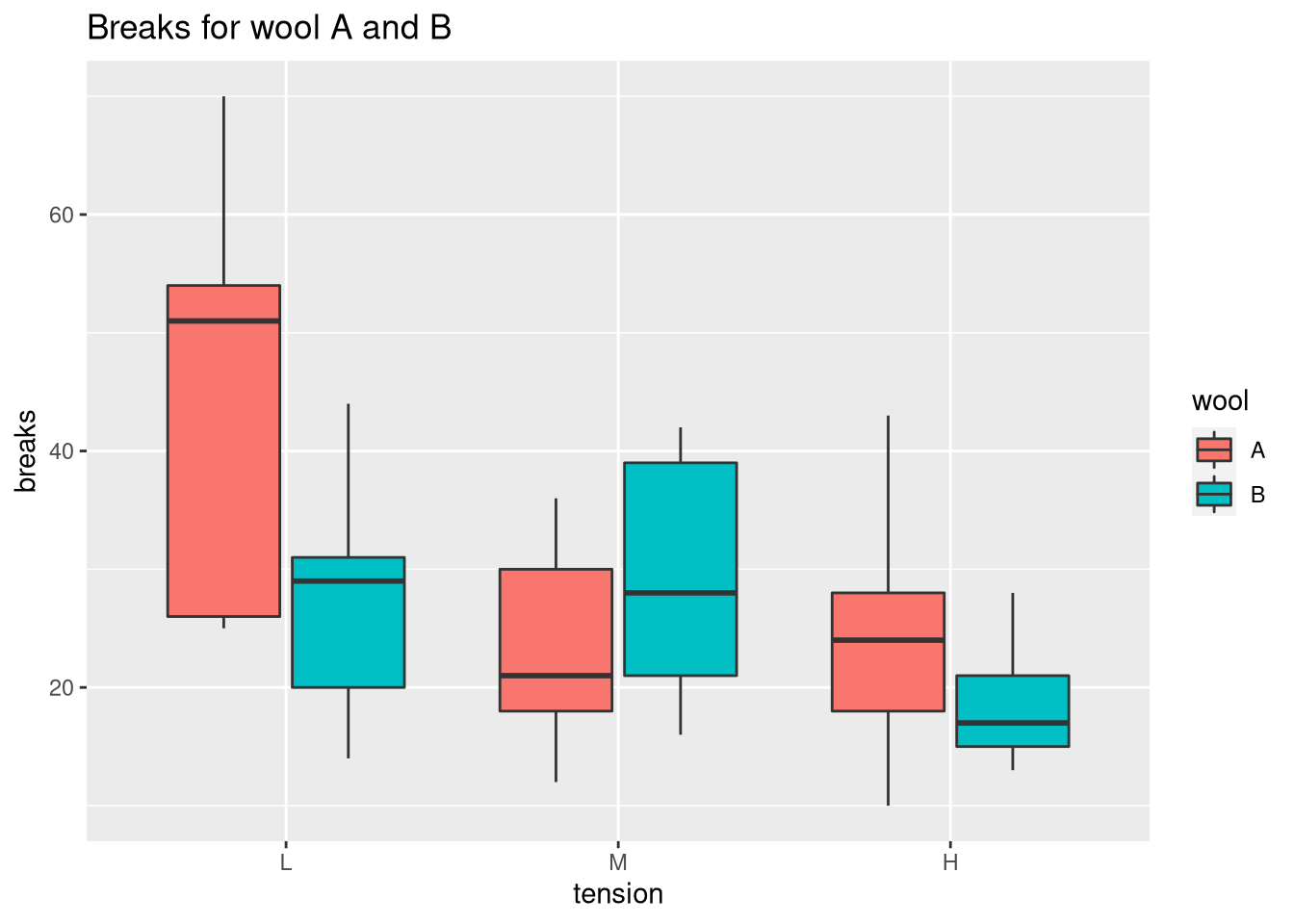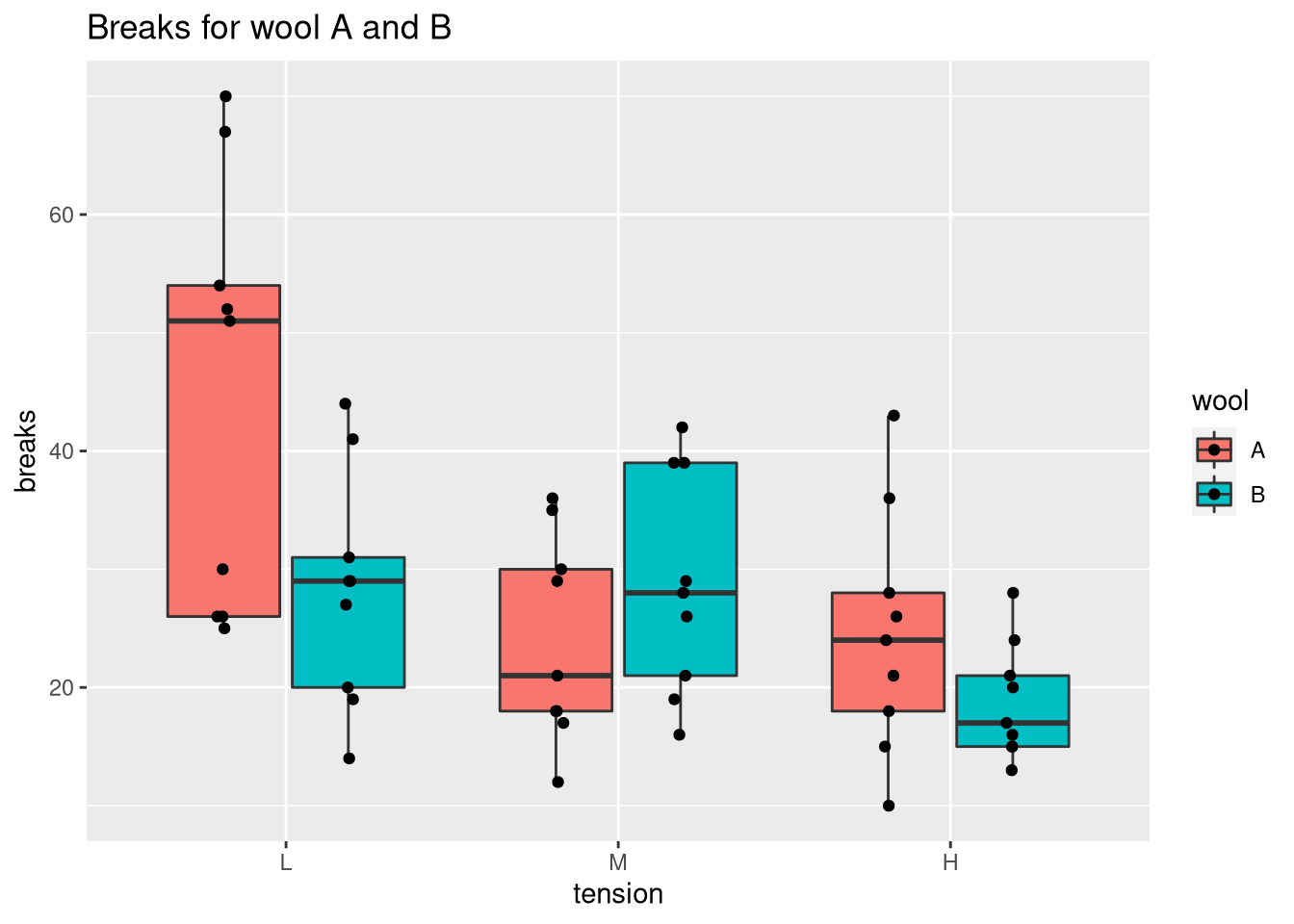# Comparing Medians and Inter-Quartile Ranges Using the Box Plot

## Creating a box plot in native R

We will use the warpbreaks data set to exemplify the use of box plots. In native R, a box plot can be obtained via boxplot.

data(warpbreaks)
# create positions for tick marks, one more than number of bars
x <- warpbreaks$breaks # create labels x.labels <- paste0(warpbreaks$wool, "-", warpbreaks$tension) # specify colors for groups group.cols <- c("darkred", "red", "darksalmon", "darkblue", "blue", "lightblue") cols <- c(rep(group.cols, 9), rep(group.cols, 9), rep(group.cols, 9), rep(group.cols, 9), rep(group.cols, 9), rep(group.cols, 9)) boxplot(x ~ warpbreaks$wool + warpbreaks\$tension, col = group.cols)
legend("topright", legend = c(unique(x.labels)),
col = group.cols, pch = 20)## Creating a box plot with ggplot

We could compare the tensions for each type of wool using facet_wrap in the following way:

library(ggplot2)
ggplot(warpbreaks, aes(x = tension, y = breaks)) +
geom_boxplot() + facet_wrap(.~wool) +
ggtitle("Breaks for wool A and B")ggplot(warpbreaks, aes(x = tension, y = breaks, fill = wool)) +
geom_boxplot() +
ggtitle("Breaks for wool A and B")### Showing all points

To view the individual measurements associated with the box plot, we set outlier.shape = NA to prevent duplicates and call geom_point.

ggplot(warpbreaks, aes(x = tension, y = breaks, fill = wool)) +
geom_boxplot(outlier.shape = NA) +
ggtitle("Breaks for wool A and B") +
# dodge points horizontally (there are two bars per tick)
# and jitter points horizontally so that they don't overlap
geom_point(position = position_jitterdodge(jitter.width = 0.1))Showing all the points helps us to identify whether the sample size is sufficient. In this case, most pairs of wool and tension exhibit high variabilities (especially wool A with tension L). Thus, the question would be whether this level of variability is inherent to the data or a result of the small number of samples (n = 9). Note that you can combine a box plot with a beeswarm plot to optimize the locations of the points.